Open in App
Not now

# Sine Rule Formula

• Last Updated : 19 May, 2022

Trigonometry is an important branch of mathematics that deals with the relation between the lengths of the sides and angles of a right-angled triangle. The word trigonometry is derived from the Greek words where ‘Tri’ means ‘three’, ‘gon’ means ‘sides’ and ‘metron’ means ‘measure’. Sine, cosine, tangent, cotangent, cosecant and secant are the six trigonometric ratios. Where a trigonometric ratio is depicted as the ratio between the sides of a triangle. Now the formulae of the trigonometric ratio are given as,

• sine θ = opposite side/hypotenuse
• cosine θ = adjacent side/hypotenuse
• tan θ = opposite side/hypotenuse
• cosecant θ = 1/sine θ = hypotenuse/opposite side
• secant θ = 1/cosine θ = hypotenuse/adjacent side
• cotangent θ = 1/tangent θ = adjacent side/opposite side

### Sine Rule or the Law of Sines

Sine Rule or the Law of Sines is a trigonometric law that gives a relationship between the sides and angles of the triangle (non-right angled triangle). Let a, b, and c be the lengths of the three sides of a triangle ABC and A, B, and C by their respective opposite angles. Now the expression for the Sine Rule is given as,

sin A/a = sin B/b = sin C/c  (or)  a/sin A = b/sin B = c/sin C

Application

By using the Sine Rule Formula we can find the length of the side of a triangle, the angle of a triangle, and also the area of a triangle.

Proof

In triangle ABC, the sides of the triangle are given by AB = c, BC = a and AC = b.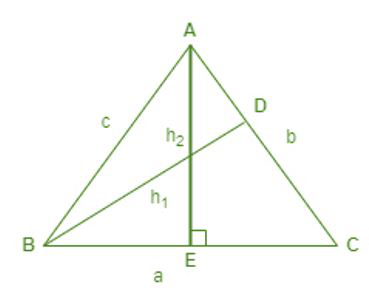Let us draw a perpendicular BD that is perpendicular to AC. Now we have two right-angles triangles ADC and BDC.

BD = h1 is the height of the triangle ABC.

sin A = h1/c ⇢ (1)

In triangle BDC,

sin C = h1/a ⇢ (2)

Now by dividing the equations (1) and (2).

We get, sin A/sin C = a/c ⇒ a/sin A = c/sin C ⇢ (3)

Similarly, draw a perpendicular AE that is perpendicular to BC. Now AEB and BEC are the right angled triangles separated by h2.

In triangle AEB,

sin B = h2/c ⇢ (4)

In triangle AEC,

sin C = h2/b ⇢ (5)

Now by dividing equations (4) and (5),

sin B/sin C = b/c ⇒ b/sin B = c/sin C ⇢ (6)

Now by equating the equations (3) and (6) we get,

a/sin A = b/sin B = c/sin C (or) sin A/a = sin B/b = sin C/c

### Sample Problems

Problem 1: Find remaining lengths of the triangle XYZ when ∠X = 30° and ∠Y = 45° and x = 5 cm.

Solution:

Given data, ∠X = 30°, ∠Y = 45° and x = 5 cm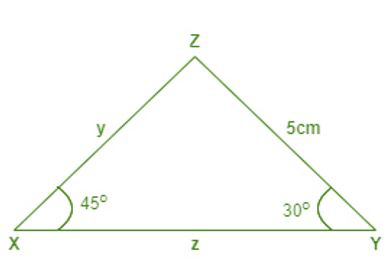We know that sum of the three angles of a triangle is 180°

So, ∠X + ∠Y + ∠Z = 1

30° + 45° + ∠Z = 180°   ⇒  75° + ∠Z = 180°

∠Z = 105°

Now from the law of Sines, x/ sin X = y/ sin Y = z/ sin Z

x/sin X = y/ sin Y ⇒ 5/ sin 30° = y/ sin 45°

⇒ x/(1/2) = y/(1/√2) ⇒ 10 =√2y ⇒ y = 7.07 cm

Similarly, x/ sin X = z/sin Z  ⇒ 5/sin 30° = z/sin 105°  [sin 105° = (√6 + √2)/4 = 0.965]

⇒ 5/(1/2) = z/(0.965) ⇒ z = 9.65 cm

Problem 2: Find ∠P and ∠Q and the length of the third side when ∠R = 36° and p = 2.5 cm and r = 7 cm?

Solution:

Given, ∠R = 36° and p = 2.5 cm and r = 7 cm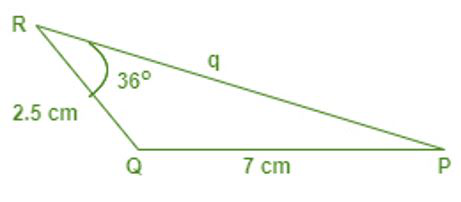From the Sine Rule Formula we have,

p/sin P = q/sin Q = r/sin R

⇒ 2.5/sin P = q/ sin Q = 7/sin 36°

⇒ 2.5/sin P = 7/sin 36° [sin 36° = 0.5878]

⇒ sin P = 0.20992 ⇒ P = sin-1 (0.20992)

⇒ ∠P = 12.12°

⇒ We have, ∠P + ∠Q + ∠R = 180°

⇒12.12° + ∠Q + 36° = 180° ⇒ ∠Q = 131.88°

⇒ q/sin 131.88° = 7/sin 36°

⇒ q/0.7445 = 7/0.5878 ⇒ q = 8.866 cm (approximately)

Hence ∠P = 12.12°, ∠Q = 131.88° and q = 8.866 cm

Problem 3: Find the ratio of the sides of the triangle ABC when ∠A = 15°, ∠B = 45°, and ∠C = 120°?

Solution:

Given: ∠A =15°, ∠B = 45° and  ∠C = 120°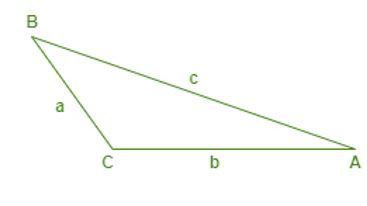From the Sine Rule formula, a/ sin A = b/ sin B = c/ sin C  ⇒  a : b : c = sin A : sin B : sin C

⇒ a : b : c = sin 15° : sin 45° : sin 120°

sin 15° = (√3 – 1)/2√2 = 0.2588 (approximate value)

sin 45° = 1/√2 = 0.7071  (approximate value)

sin 120° = √3/2 = 0.866  (approximate value)

Hence the ratio of the three sides of the triangle ABC is a : b : c = 0.2588 : 0.7071 : 0.866

Problem 4: Find the area of the triangle ABC when BC = 10 cm, AB = 12 cm and ∠B= 30°?

Solution:

Given, BC = a = 10 cm, AB = c = 12 cm and ∠B= 30°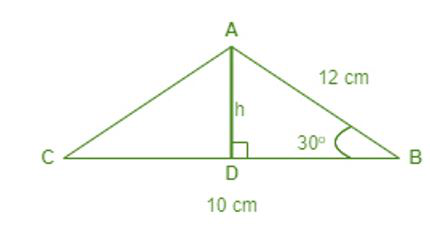We know that, Area of the triangle = ½ (base) (height) = ½ (a) (h) ⇢ (1)

From the figure, sin B = height/c

h = c sin B ⇢ (2)

Now substitute equation (2) in (1),

Area of the triangle ABC = ½ (a)(c) sin B= ½ (10) (12) sin 30° [sin 30° = ½]

⇒ Area = ½ (120) ½ = 30 cm2

Hence the area of the triangle ABC is 30 cm2.

Problem 5: Find ∠ACB if a = 3 cm, c = 1 cm, and ∠BAC = 60°.

Solution:

Given, ∠BAC = 60°, a = 3 cm and c = 1 cm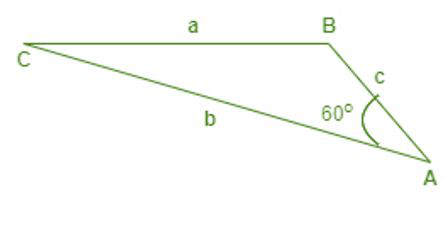From the Sine Rule Formula, we have sin A/a = sin C/c

⇒ sin 60°/3 = sin C/1   [sin 60° = √3/2]

⇒ (√3/2)/3 = sin C/3 ⇒ sin C = 1/(2√3) ⇒ sin C = 0.2887 (approximately)

⇒ ∠C = sin-1(0.2887) ⇒  ∠C = 16.77°

Hence ∠ACB = 16.77°

Problem 6: Find the length of the side YZ, if the area of the triangle XYZ is 24 cm2, ∠Y = 45°, and z = 4 cm.

Solution:

Given, Area of the triangle XYZ = 24 cm2, ∠y = 45° and z = 4 cm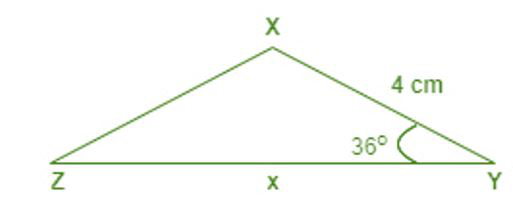From the Sine rule law, we have Area of triangle XYZ = ½ (x)(z) sin Y

⇒ 24 = ½ (x)(4) sin 45°       [sin 45° = 1/√2]

⇒ 24 = (x) × (2) × (1/√2)

⇒ 12√2 = x  ⇒ x = 16.968 cm

Hence the length of the side YZ = x = 16.968 cm

Problem 7: Find q  when ∠P= 108°, ∠Q = 43°and p = 15 cm?

Solution:

Given, ∠P = 108° , ∠Q = 43° and p = 15 cm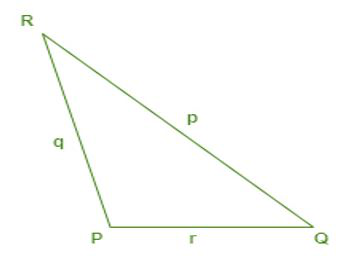From the Sine Rule Formula we have, p/sin P = q/sin Q

⇒ 15/sin 108° = q/sin 43°

⇒ 15/(0.9510) = q/(0.6820) [sin 108° = 0.9510 & sin 43° = 0.6820]

⇒ q = 10.757 cm (approximately)

Hence q =10.57 cm

My Personal Notes arrow_drop_up